## python处理excel有哪些类库

`xlrd``xlwt``openpyxl`都是python常用的处理excel类库

• xlrd 是只能读取excel文档内容
• xlwt 是只能将内容数据写入excel文档 xlrd和xlwt这两个库结合起来功能也很丰富，不过今天我们一起来了解下openpyxl类库，openpyxl库既可以读excel文档，也可以将数据写入excel文档，并且还可以操作sheet表格，生成图表（比如柱状图、饼图)等。

## 搭建openpyxl类库环境

``````pip install openpyxl
``````

``````pip install openpyxl -i https://mirrors.aliyun.com/pypi/simple/
``````

## 了解下excel的基本概念

• 工作薄 （workbook），我们通俗说的一个excel文档
• 工作表 （sheet），一个工作薄最少包含一个工作表，可以有多个，我们通常看到excel下面显示的`sheet1``sheet2``sheet3`等，当然我们也可以对工作表重命名
• 列 （column），也就是工作表中竖着的列，从A开始记录位置
• 行 （row），工作表中横向的行，从1开始记录位置
• 单元格 （cell），工作表中一个一个的表格，并且位置由列和行的位置标号记录（比如：A1，C5）

## openpyxl简单操作excel的三个基本步骤

1. 加载workbook到内存
2. 打开要处理的sheet
3. 读写单元格cell

``````from openpyxl import load_workbook
``````

``````#相对路径，与当前程序在同一目录下

#绝对路径，可以指向计算机上任何能访问到的位置
``````

## 3、打开指定的sheet表格

``````#打开工作表有两种方式：
#第一种：通过工作表名称打开工作表
sheet=wb["sheet1"]
#第二种：获取活跃的工作表，也就是默认打开显示的表格
sheet=wb.active    #['sheet1']
``````

``````#获取所有的工作表
wb.sheetnames    #['sheet1']
#修改工作表名称
sheet.title="students"
#获取工作表名称
sheet.title    #students
``````

## 4、单元格常用操作

``````sheet.cell(2,3)    #<Cell 'students'.C2>
sheet["C2"]    #<Cell 'students'.C2>
``````

``````#方式一
sheet.cell(2,3).value    #60
#方式二
sheet["C2"].value    #60
``````

``````#方式一
sheet.cell(2,4).value="及格"
#方式二
sheet["D3"]="及格"
#方式三
sheet.cell(4,4,"良好")
#保存工作簿
wb.save("学生表.xlsx")
``````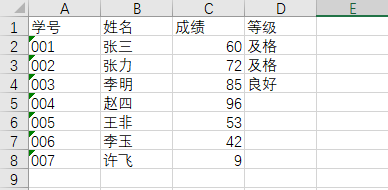``````#获取最大行：
sheet.max_row    #8
#获取最大列：
sheet.max_column    #4
``````

``````#获取A1到A3的单元格
cell1=sheet['A1:A3']
#获取第一行和第二行的单元格
cell2=sheet[1:2]
#获取A列到C列的单元格
cell3=sheet['A:C']
``````

``````#获取行数1-2且列数1-4的单元格，逐行读取
cell4=sheet.iter_rows(min_row=1, max_row=2, min_col=1, max_col=4)
for cell in cell4:
print(cell)
# 上面获取的是单元格，如果想得到单元格的值，只需加个参数
cell4=sheet.iter_rows(min_row=1, max_row=2, min_col=1, max_col=4,values_only=True)
#获取列数1-4且行数1-2的单元格，逐列读取
cell5=sheet.iter_cols(min_col=1, max_col=4,min_row=1, max_row=2)
for cell in cell5:
print(cell)
#同样，想获取单元格的值，加参数
cell5=sheet.iter_cols(min_col=1, max_col=4,min_row=1, max_row=2,values_only=True)
``````

## 5、插入/删除行、列

``````#插入行
sheet.insert_rows(idx=数字编号,amount=要插入行数)
#删除行
sheet.delete_rows(idx=数字编号,amount=要插入行数)
``````
``````#从第二行开始插入三行
sheet.insert_rows(idx=2,amount=3
``````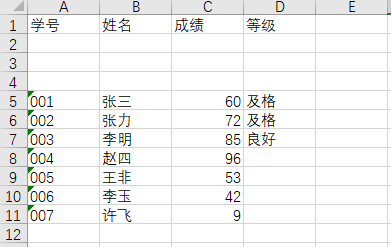``````#第2行开始删除3行
sheet.delete_rows(idx=2,amount=3)
#插入列
sheet.insert_cols(idx=数字编号,amount=要插入列数)
#删除列
sheet.delete_cols(idx=数字编号,amount=要插入列数)
``````

## 生成折线图和柱状图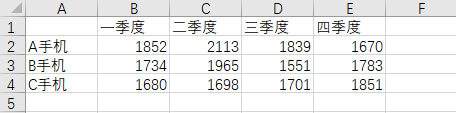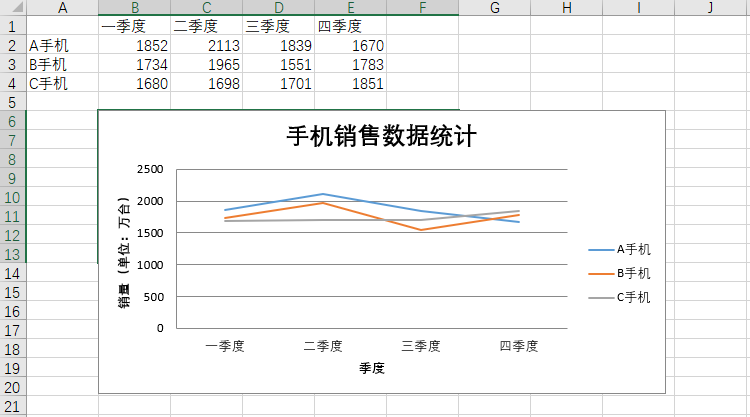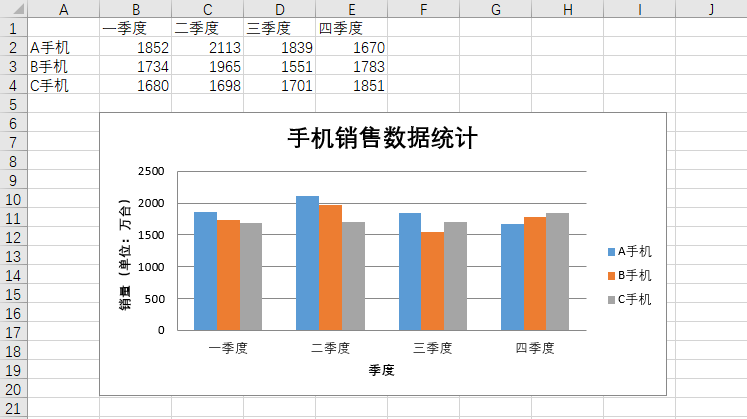``````from openpyxl import load_workbook
# 生成折线图
from openpyxl.chart import LineChart,Reference
sheet=wb.active
chart=LineChart()
# 图的标题
chart.title="手机销售数据统计"
# y轴标题
chart.y_axis.title="销量（单位：万台）"
# x轴标题
chart.x_axis.title="季度"
# 数据来源
data=Reference(worksheet=sheet,min_row=2,max_row=4,min_col=1,max_col=5)
# 设定X轴项目名称,项目名称来自第一行的第二列至第五行
categories=Reference(sheet,min_col=2,min_row=1,max_col=5,max_row=1)
# 给折线图添加数据，数据源中有系列名称，系列名称来自行，第一行为系列名称
chart.set_categories(categories)
wb.save("销售数据.xlsx")
``````
``````from openpyxl import load_workbook
#生成柱状图
from openpyxl.chart import BarChart,Reference
sheet=wb.active
bc=BarChart()
# 图的标题
bc.title="手机销售数据统计"
# y轴标题
bc.y_axis.title="销量（单位：万台）"
# x轴标题
bc.x_axis.title="季度"
# 数据来源
bc_data=Reference(worksheet=sheet,min_row=2,max_row=4,min_col=1,max_col=5)
# 设定X轴项目名称,项目名称来自第一行的第二列至第五行
bc_cat=Reference(sheet,min_col=2,min_row=1,max_col=5,max_row=1)
# 给柱状图添加数据，数据源中有系列名称，系列名称来自行，第一行为系列名称